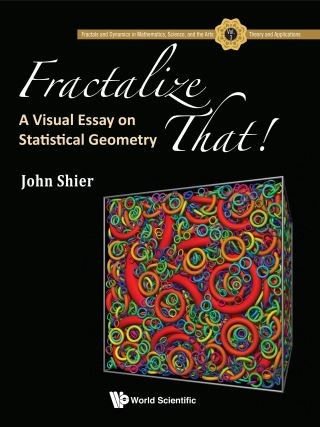## Fractalize That!• Description
• Author
• Info
• Reviews

### Description

Fractalize That! A Visual Essay on Statistical Geometry brings a new class of geometric fractals to a wider audience of mathematicians and scientists. It describes a recently discovered random fractal space-filling algorithm. Connections with tessellations and known fractals such as Sierpinski are developed. And, the mathematical development is illustrated by a large number of colorful images that will charm the readers.

The algorithm claims to be universal in scope, in that it can fill any spatial region with smaller and smaller fill regions of any shape. The filling is complete in the limit of an infinite number of fill regions. This book presents a descriptive development of the subject using the traditional shapes of geometry such as discs, squares, and triangles. It contains a detailed mathematical treatment of all that is currently known about the algorithm, as well as a chapter on software implementation of the algorithm.

The mathematician will find a wealth of interesting conjectures supported by numerical computation. Physicists are offered a model looking for an application. The patterns generated are often quite interesting as abstract art. Readers can also create these computer-generated art with the advice and examples provided.

Contents:
• Introduction: Space-Filling Patterns
• Squares and Rectangles
• Triangles and Diamonds
• Discs and Circular Arcs
• Mixed and Irregular Shapes
• Examples
• The Statistical Geometry Algorithm
• The Area–Perimeter Algorithm
• Statistical Geometry in One Dimension
• Do It Yourself

Readership: Students, mathematicians, physicists, professionals, and the general public who are interested in the fractal art of statistical geometry.
Key Features:
• The book brings together information from the few published papers and unpublished material reported here for the first time
• This is the first book-length description of the subject
• Examples of the use of the algorithm for decorative art
• A visual feast